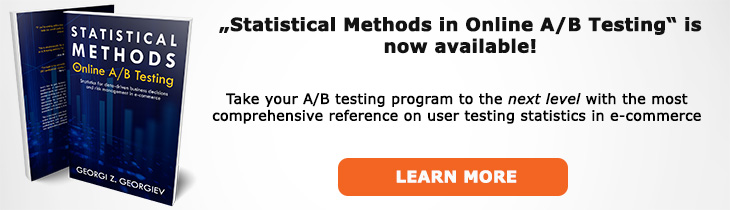# What does "Minimum Effect of Interest" mean?

Definition of Minimum Effect of Interest in the context of A/B testing (online controlled experiments).

## What is a Minimum Effect of Interest?

Aliases: MEI

The minimum effect of interest is the effect size we would be happy/excited to find by using a statistical test to analyze a randomized controlled experiment, a.k.a. an A/B test. It is usually denoted μ1.The choice of a proper MEI is vital for setting the sample size of the experiment so that it provides sufficient statistical power at the point of the MEI. Once a significance threshold, power level and a minimum effect of interest are chosen, the sample size is then fixed.

However, choosing a minimum effect of interest is not straightforward as it results in a feedback loop involving the sample size and therefore test duration and the significance threshold. For example, a lift of 1% might be exciting if the number of users per week mean we can detect it with probability 90% (90% statistical power) in 6 weeks, but the same 1% lift might be much less exciting if we would need the test to run for 50 or 60 weeks to achieve the same power level. In such cases the MEI or the significance threshold need to be altered, or both, until a suitable compromise is found. How much the minimum effect of interest can shift is, however, limited by the range of probable effect sizes one expects based on the type and magnitude of the tested treatment. For example, even though a MEI of 10% might be preferred due to the shorter test duration, if the range of the expected effect size spans -5%..5% then it is nearly futile to run such a test as it will have very low probability of a true positive and a very high probability of a false negative.

In many cases an optimal sample size and significance threshold can be approximately calculated using Risk-Reward Analysis which includes Power Analysis as a sub-task. Then the power function shows the minimum detectable effect (MDE) at different power levels and so an optimal minimum effect of interest can be determined based on the desired power level.

Sometimes the term "Minimum detectable effect" (MDE) is used instead of MEI, but this can be confusing since it refers to a technical characteristic of the statistical test instead of an input parameter for such a test. Its wording also hints that true effects below it would not be detected which is not the case and this can easily be established by examining the power function (which has a value of alpha (α) at the point of the null hypothesis closest to the alternative hypothesis). The term "minimum effect of interest" should be preferred when talking about an input parameter for a test design since it reflects the fact that it is the minimum effect we want to detect reliably and does not carry with it the possibility of confusing it with a minimum effect size which can produce a statistically significant outcome which is a completely different quantity.

## Related A/B Testing terms

Statistical Power

Effect Size

Minimum Detectable Effect

## Articles on Minimum Effect of Interest

Like this glossary entry? For an in-depth and comprehensive reading on A/B testing stats, check out the book "Statistical Methods in Online A/B Testing" by the author of this glossary, Georgi Georgiev.## Glossary Index by Letter

Select a letter to see all A/B testing terms starting with that letter or visit the Glossary homepage to see all.